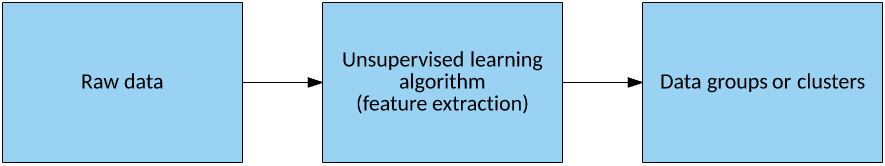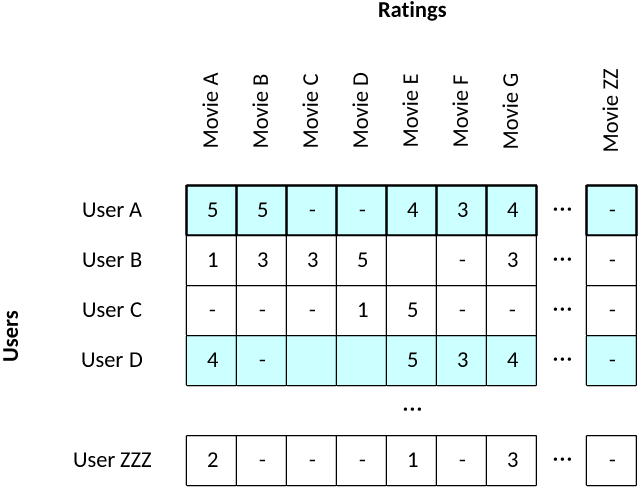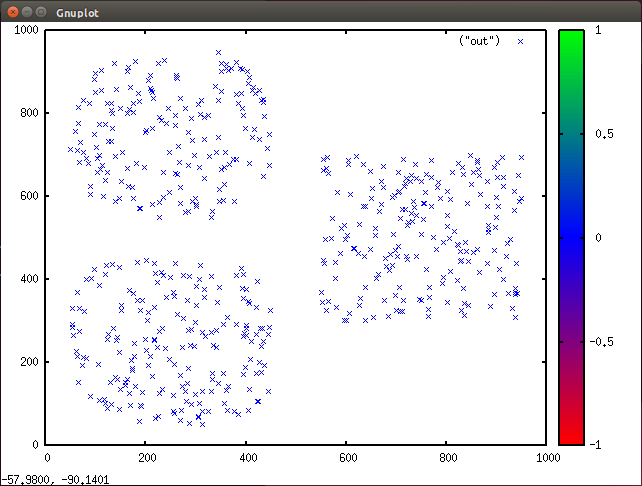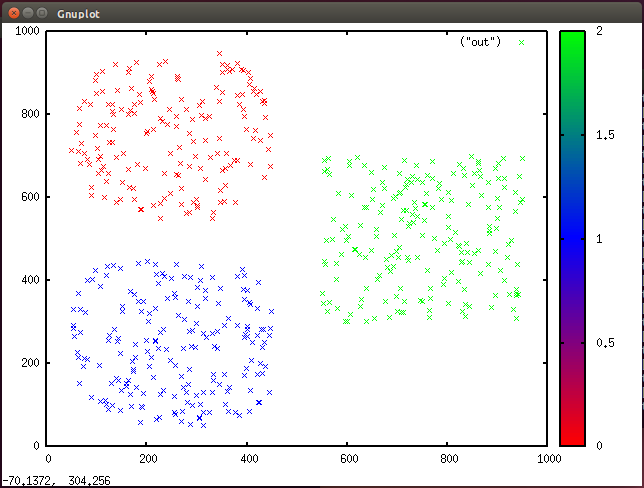Unsupervised learning for data classification

In unsupervised learning, an algorithm segregates the data in a data set in which the data is unlabeled based on some hidden features in the data. This function can be useful for discovering the hidden structure of data and for tasks like anomaly detection. This tutorial explains the ideas behind unsupervised learning and its applications, and then illustrates these ideas in the context of exploring data.

Unsupervised learning algorithms group the data in an unlabeled data set based on the underlying hidden features in the data (see Figure 1). Because there are no labels, there’s no way to evaluate the result (a key difference of supervised learning algorithms). By grouping data through unsupervised learning, you learn something about the raw data that likely wasn’t visible otherwise. In highly dimensional or large data sets, this problem is even more pronounced.

Figure 1. Unsupervised learning algorithmIn addition to clustering data into groups, the algorithm makes it possible to use these groups to exploit the hidden features.

Feature vectors

Consider a data set that lists movies by user rating. Users assign a rating to each movie watched from 1 – 5 (1 being bad, 5 being good). Each user is represented by a feature vector that contains the movie ratings that user provided. By clustering the users into groups, you can find people who have similar movie interests or similar dislikes (see Figure 2). By considering a cluster, you can find differences in the feature vectors that might be suitable for recommendation (a movie common in the cluster that some members of the cluster might have not yet seen).

Figure 2. A simple feature vector set for movie ratingConsider users A and D. They have similar tastes in movies based on their ratings and would likely be part of the same cluster of users. From Figure 2, you can see that user A enjoyed “Movie B,” but user D hasn’t rated that one, yet. Therefore, “Movie B” appears to be a good recommendation to user D based on his or her similar tastes (and secondarily, his or her differences).

Note here that the feature vector can be large. For example, Amazon Prime has just over 20,000 titles. One solution to this problem is dimensionality reduction to reduce the number of features under consideration. Using the existing example, you could manually reduce the size of your feature vector by representing not the individual ratings themselves but the sum or average of the ratings for a given genre or title (cyberpunk, spaghetti western, or Japanese horror). Techniques exist to reduce dimensionality by using computational methods such as principal component analysis.

This is one contrived example of how you could use clustering to recommend content to users based on their cluster membership and the differences in their feature vectors. Now, let’s dig into some of the methods that are used for unsupervised learning.

Methods for clustering

A popular algorithm for clustering data is the Adaptive Resonance Theory (ART) family of algorithms—a set of neural network models that you can use for pattern recognition and prediction. The ART1 algorithm maps an input vector to a neuron in a recognition field through a weight vector. A key advantage of ART is that it incorporates a vigilance parameter to determine how closely a feature vector must map to a cluster for it to be a member. Otherwise, it can create a new cluster automatically for the feature vector. The vigilance parameter dictates whether there are many clusters that more closely match the input vectors (high vigilance) or general clusters that incorporate more feature vector examples (low vigilance).

Another neural network method, called self-organizing map (SOM), translates multidimensional feature vectors into a low-dimensional representation called a map. This method implements a form of data compression through vector quantization. The neural network makes up the map, which is typically a regular lattice shape (NxM neurons representing a rectangular grid). Each neuron in the map has a weight vector that matches the dimensionality of the input vector (as shown in the example in Figure 3, 2).

Figure 3. A typical self-organizing mapThe input vector connects to each neuron in the map. During training, each observation is applied to the input vector, and the Euclidean distance of the observation is calculated for each weight vector of the map. The neuron whose weight vector is closest to the input vector (minimal distance) is declared the winner, and the weights are updated toward the input vector. This process continues for each observation in the data set. When training is complete, a new observation can be applied to the network, and the winning neuron represents its cluster.

One of the simplest and most effective algorithms for clustering data is the k-means algorithm. SOMs are similar to k-means (for a small number of neurons), but SOMs have an advantage in that the map is topological in nature and can be easy to visualize, even for highly dimensional data. Let’s explore the k-means algorithm and its implementation for a simple data set.

k-Means clustering

The ideas behind k-means clustering are simple to visualize and understand. The k-means algorithm organizes a set of observations that are represented as feature vectors into clusters based on their similarity. Their similarity is in turn based on a distance metric of k centroids (the centroid being the center of a cluster based on the mean of that cluster’s members). The Euclidean distance is used as the similarity metric; the closest centroid (also represented as a feature vector) is the cluster in which a feature vector should be a member.

Now let’s look at the training algorithm for k-means. There are three basic steps—Initialization, Update, and Assignment—the first of which has a considerable variability. For initialization, I’ll demonstrate what’s called random partition, which assigns each observation from the data set randomly to one of the k clusters. Another method, called the Forgy method, simply takes k observations randomly from the data set and assigns each to a cluster (see Figure 4).

Figure 4. A typical k-means clustering algorithmThe update step calculates the centroids based on the observations part of the cluster, which is simply the mean of all feature vectors that are part of the cluster.

With the centroids updated, I next take the Assignment step. In this step, I look at each observation and test whether it’s part of the correct cluster. I do this by calculating the Euclidean distance of the observation’s feature vector with each k centroid. The closest cluster represents the cluster of which the observation should be part. If that cluster is different from the observation’s current cluster, then the observation is moved. If I exit the Assignment step without any observations having changed cluster, then the algorithm is converged and I’m done. Otherwise, I return to the update step to update the centroids and begin again.

Let’s look at a straightforward implementation of k-means that I’ll use to cluster a simple data set.

k-Means implementation

You can find this C language implementation at GitHub for the Linux operating system. I’ll explore this implementation in a top-down fashion, starting with the main, and then digging into the initialization and update steps through their respective functions.

When you build the sample implementation, you get two executables results. The first is called gendata, and you can use this utility to generate a random data set of approximately 600 observations. Each observation represents a feature vector of two attributes (x and y location in two-dimensional space). This data set is contrived so that it’s easy to see where the clusters should be formed for k = 3, as shown in Figure 5 (plotted with Gnuplot).

Figure 5. A scatter plot of clusters generated in GnuplotThe second executable provided is called kmeans. You can use this executable to cluster the test data.

Observations and centroids are represented as structure arrays in C, where a centroid is represented as two attributes and an observation is represented by the feature vector (two attributes) and the class to which it belongs:

typedef struct observation
{
int    x;
int    y;
int class;
} observation;

typedef struct centroid
{
double x;
double y;
} centroid;

#define K                3
#define MAX_SAMPLES    600

centroid Centroids[ K ];
observation Observations[ MAX_SAMPLES ];

The main function serves as the main loop for the k-means algorithm. After reading the data set through a call to Read_Dataset, I assign the observations to a random cluster, and then perform the Update and Assignment steps until no cluster membership changes occur. I then write the data set out so that it can be plotted:

int main( int argc, char *argv[] )
{
int Samples;

if ( argc != 2 )
{
printf( "Usage is %s <observations_file>\n\n", argv );
return 0;
}

srand( time( NULL ) );

// Read the input dataset from the file.

// Initialization step, initialize observations to clusters.
Initialization( Samples );

// Update step, continue to perform until convergence.
do
{
Update( Samples );
}
while ( Assignment( Samples ) );

// Write the dataset back out.
Write_Dataset( Samples );

return 0;
}

In the Initialization function, I randomly assign the observations to one of the three clusters:

void Initialization( int Samples )
{
// Assign each observation to a random cluster.
for ( int Target = 0 ; Target < Samples ; Target++ )
{
Observations[ Target ].class = getRand( K );
}

return;
}

The Assignment function is also quite simple. I iterate through each observation to find the cluster to which it belongs. If the cluster changes for a given observation, I keep track of this to identify how many observations moved for a given assignment step:

int Assignment( int Samples )
{
int Moved = 0;

for ( int Index = 0 ; Index < Samples ; Index++ )
{
int NearestClass = FindNearestCluster( Index );

if ( NearestClass != Observations[ Index ].class )
{
// Change the membership of this observation
// to the new cluster.
Observations[ Index ].class = NearestClass;
Moved++;
}

}

return Moved;
}

To update the centroids (the center of the cluster), the Update function first clears out the cluster centroid and the number of observations associated with it (MembershipCount). Next, each observation is checked, and the feature vector is simply summed into the centroid to which it belongs. The average is then calculated by dividing by the number of observations associated with that cluster:

void Update( int Samples )
{
int MembershipCount[ K ];

// Initialize the clusters
for ( int Cluster = 0 ; Cluster < K ; Cluster++ )
{
Centroids[ Cluster ].x = 0.0;
Centroids[ Cluster ].y = 0.0;
MembershipCount[ Cluster ] = 0;
}

// Update the cluster centroids
for ( int Index = 0 ; Index < Samples ; Index++ )
{
Centroids[ Observations[ Index ].class ].x +=
(double)Observations[ Index ].x;
Centroids[ Observations[ Index ].class ].y +=
(double)Observations[ Index ].y;
MembershipCount[ Observations[ Index ].class ]++;
}

// Update centroids to average based upon the number of members.
for ( int Cluster = 0 ; Cluster < K ; Cluster++ )
{
if ( MembershipCount[ Cluster ] > 1 )
{
Centroids[ Cluster ].x /=
(double)MembershipCount[ Cluster ];
Centroids[ Cluster ].y /=
(double)MembershipCount[ Cluster ];
}
}

return;
}

The final two functions implement the Euclidean distance for two points (distance), and FindNearestCluster implements a simple test whereby the distance between a given observation is calculated for cluster 0, and then clusters 1 and 2 are checked to see whether the distance decreases (to determine the closest centroid for the observation):

double distance( int x1, int y1, int x2, int y2 )
{
return sqrt( SQR( ( x2 ‑ x1 ) ) + SQR( ( y2 ‑ y1 ) ) );
}

int FindNearestCluster( int Index )
{
int    NearestCluster;
double Closest;

// Initialize with the first cluster
NearestCluster = 0;
Closest = distance( (double)Observations[ Index ].x,
(double)Observations[ Index ].y,
Centroids[ NearestCluster ].x,
Centroids[ NearestCluster ].y );

// Now test against the other K‑1
for ( int Cluster = 1 ; Cluster < K ; Cluster++ )
{
double Distance;
Distance = distance( (double)Observations[ Index ].x,
(double)Observations[ Index ].y,
Centroids[ Cluster ].x,
Centroids[ Cluster ].y );

if ( Distance < Closest )
{
Closest = Distance;
NearestCluster = Cluster;
}
}

return NearestCluster;
}

The output written from Write_Dataset includes the attributes from the feature vector and the cluster to which it belongs. Plotting this data with color assignments for each cluster results in the graph in Figure 6 (using Gnuplot).

Figure 6. Clustered data with color assignments in Gnuplot (three clusters)Note here that the data set was created with three clusters in mind. If I update k in my program to 6, it identifies six separate clusters, although three was the natural decomposition (see Figure 7).

Figure 7. Clustered data with color assignments in Gnuplot (six clusters)Other cluster algorithms (such as ART) can automatically identify the proper number of clusters based on the data. This is one of the shortcomings of k-means.

Using the programs

You can use the sample programs to generate a test data set, and then cluster the data set by using k-means. The following commands demonstrate how to build the sample programs, generate the test data, cluster it, and plot the result with Gnuplot:

\$ make
\$ ./gendata > test.data
\$ ./kmeans test.dat > clustered.data
\$ gnuplot

gnuplot> set terminal x11
gnuplot> set palette model RGB defined (0 "red", 1 "blue", 2 "green")
gnuplot> set xrange0:1000gnuplot> set yrange0:1000gnuplot> plot ("clustered.data") with points pt 2 palette

Going further

Unsupervised learning is a useful technique for clustering data when your data set lacks labels. Once clustered, you can further study the data set to identify hidden features of that data. This tutorial discussed ART and SOM, and then demonstrated clustering by using the k-means algorithm. You’ll find clustering algorithms like these in use in a variety of applications, most recently in security for anomaly detection.

Ready to go deeper? Access code patterns and learn how to hook it all together. Take it to the next step by learning how to classify art using TensorFlow.

Legend